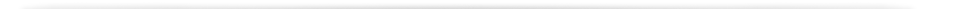# Chromatography Conversion Calculators

## Chromatography Conversion Calculators

### What do you want to calculate ?

Calculation is made from the following formula:
Bed volume (L) = bed height (cm) x column crossectional area (cm2) / 1000

Bed Height (cm)
Column Diameter (cm)
Calculate Bed Volume

### Bed Volume

0.00
Liters ResetCalculation is made from the following formula:
Residence time (min) = [bed height (cm) / flow velocity (cm/h)] x 60

Flow Velocity (cm/hr)
Bed Height (cm)
Calculate Residence Time

### Residence Time

0.00
Minutes ResetCalculation is made from the following formula:
Volumetric flow rate (L/h) = (flow velocity (cm/h) x (column crossectional area (cm2) / 1000).

Flow Velocity (cm/hr)
Column Diameter (cm)
Convert Flow Rate

### Volumetric Flow Rate

0.00
Liters Per Hour

0.00
Milliliters Per Minute
ResetCeramic Apatite Type
Column ID (cm)
Resin Bed Height (cm)
Slurry Percentage (%v/v)
Recommendation: If manually pouring resin into the column: 30–50%. If pumping resin into the column: 20–25%.
Packing Density (gm/cm3)
The default value is the recommended packing density for this resin, or enter a different preferred packing density.
Calculate

0.00
Kilograms

### Volume of Buffer

0.00
Liters Reset